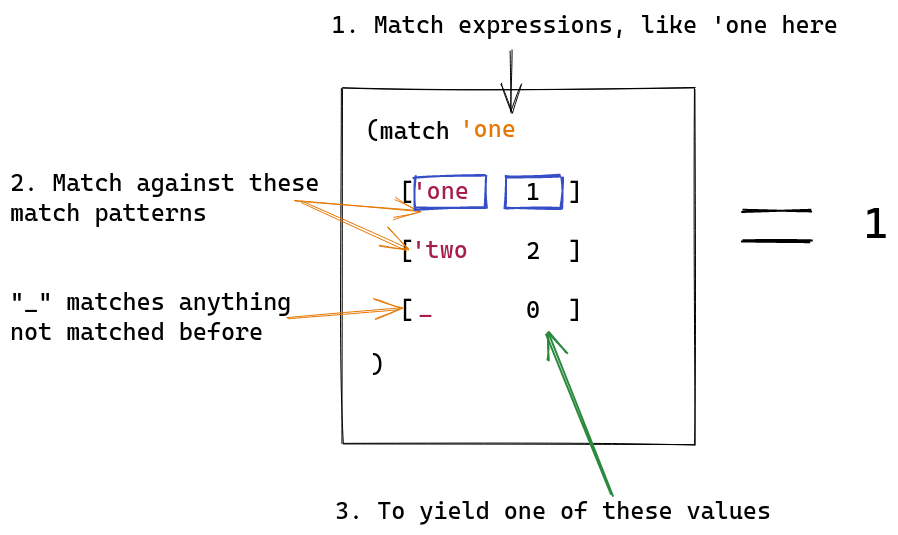## Macros and metaprogramming

Macros are procedures that re-write code at compile time. Macros are a form of metaprogramming. Metaprogramming sounds really hard to understand, but it’s really just a program which can write other programs.

### A metaprogramming example in Python

Here’s a quick example using Python, the language I’m most familiar with. It takes a number and generates code which prints every number from `0..number`.

``````#!/usr/bin/env python

import sys

if __name__ == '__main__':
if len(sys.argv) < 2:
print("Usage: code-generate.py [number]")
sys.exit(1)

number = int(sys.argv)
out = [
f"print({num})"
for num in range(0, number)
]

print("; ".join(out))
``````

You can then pass the output of this program to Python and run it.

``````\$ python -c "\$(python code-generate.py 4)"
0
1
2
3
``````

## Macros in Racket

Many languages have macros, but today I will be exploring how to use them in Racket.

To understand macros in Racket, there are two key concepts I needed to learn about first: Syntax objects and pattern matching.

### Syntax objects

In the example I gave above in Python, I used a string template to create new code.

In Racket, macros accept and return something called a syntax object.

A syntax object is a piece of data which contains everything Racket needs to know about a piece of code in order to execute it, including:

• The literal code which is represented by the syntax object
• The location (line and column) and filename represented by the syntax object
• Optionally, data stored in syntax properties. I’m not sure what these are used for yet.
• Optionally, other syntax objects

Syntax objects can be instantiated using two forms, one is by using `syntax` and the other is by using the syntax prefix `#'`. Syntax can be inspected with the `syntax->datum` procedure.

``````> (define stx (syntax (+ 1 2)))
> (define stx2 #'(+ 1 2))
> (syntax->datum stx)
'(+ 1 2)
> (syntax->datum stx2)
'(+ 1 2)
``````

For simplicity, we can think of syntax objects as just containers of code that can be manipulated like any other variable. We will be doing some manipulation of this code to produce other containers of code which are larger than the original.

### Pattern matching

Pattern matching is the ability to match a pattern in code and do something useful with it.

In JavaScript, we can do pattern matching with a destructuring assignment:

``````> const [_, second, ...rest] = [1, 2, 3, 4, 5]
undefined
> console.log(`second: \${second} rest: \${rest}`)
second: 2 rest: 3,4,5
> const { a } = { a: 'hey!' }
undefined
> console.log(`a: \${a}`)
a: hey!
``````

We can do similar things in Racket, using `match`:

``````> (match 'one
['one 1]
['two 2]
[_ 0]
)
1
``````

Here’s an illustration I created to help me understand this:In the above example, `match 1` is called the “target expression” and the other lines like `[1 "one"]` are called the “pattern expression.” Lines in the pattern expression are laid out like this: `[pattern-to-match-against pattern-expression]`.

We can also bind variables in the target expression to variables in the pattern expression, allowing us to perform more complex matches.

``````> (match '(5 5)
[(list x y) (+ x y)]
[(cons x y) (* y x)]
)
10
> (match '(5 . 5)
[(list x y) (+ x y)]
[(cons x y) (* y x)]
)
25
``````

Macros don’t use this exact syntax, but they use something really similar. Knowing how pattern matching, and particularly how the value binding works, is crucial to understand macros.

### Pattern matching on syntax objects

The first type of macros described in the Racket Guide are Pattern-Based Macros. These macros do the following:

1. Accept a syntax object and match parts of it against a pattern
2. Bind the matched parts to variables
3. Uses the variables from step 2 in something called a template, which expands the matched code

One of the ways to define a macro is to use `define-syntax` and `syntax-rules`. Here `define-syntax` defines the name or `id` of the macro we are creating, and `syntax-rules` is where we put the pattern matching.

#### My first macro

I’m going to try to make a `for..in` loop macro using `define-syntax` and `syntax-rules`. I got the idea from the article Hygenic and referentially-transparent macros with syntax-rules.

Here’s what I hope to be able to compile and run once this is complete:

``````> (for item in '(1 2 3 4) (begin
(display item)
(newline)
))
1
2
3
4
``````

Here’s the code I ended up getting to compile. It doesn’t quite work as I expect it too, so that will be something I look into tomorrow:

``````#lang racket

(define-syntax for
(syntax-rules (in)  ; needed to match against for in
[(for item in list body ...)
(letrec ([for-in-helper (lambda (l)
(cond
((empty? l) '())
(else
(let ([item (car l)])
(cons body ... (for-in-helper (cdr l)))))))])
(for-in-helper list))]))

(let ([list '(1 2 3 4)])
(for item in list (begin
(display item)
(newline))))
``````

But it compiles!